# Taking the Fun Out of Puzzles & Games

## Monday, December 30, 2013

### Mentally calculating the # of Tuesdays between 1700 and 2014

This note explains one way to calculate, in your head, the number of Tuesdays between Jan 1, 1700 and Jan 1, 2014. The overall approach is to calculate how many days there were in the years 1700, 1701, 1702, ..., 2014, and then divide by 7 to get the number of weeks, which gives the number of Tuesdays.

In this note I will talk about some mental tricks/techniques we can use for this, including:

Let's begin. Overall, there are 2014 - 1700 = 314 years from 1700 to 2014. This works out to 365·314 + L days, where L is the number of leap years in that period.

The first step is to multiply 365·314. We can simplify this with some basic algebra, by noting that (a+b)(a+c) = a(a + b + c) + bc. Letting a = 300, b = 65, and c = 14, we have: 365·314 = (300 + 65)(300 + 14) = 300(300 + 65 + 14) + (65·14)
= 300·379 + 65·14.

300·379 isn't too bad to do in your head; it's 900 + 210 + 27 = 1137, and then adding two zeros gives 113700. We will need to remember this number for later. Using the mnemonic major system we can remember the first four digits 1137 as "toot mic" (picture someone blowing a horn into a microphone).

Now for 65·14. By writing this as 66·14 - 14, we can use the algebra from above and set a = (66+14)/2 = 40, b = 26, and c = -26, which gives us 66·14 = (40 + 26)(40 - 26) = 402 - 262, a difference of two squares. There are many tricks for doing squares.  One is that ab^2 = 100a2 + 10·2ab + b2, so 262 = 100·4 + 10·24 + 36 = 400 + 240 + 36 = 676, so 402 - 262 works out to 1600-676. It is often simpler to calculate x - y by converting it to x + ~y, where ~y is the "second complement" of y. Here the second complement of 676 is 324 - 1000, so we have 1600 - 676 = 1600 + (324 - 1000) = 600 + 324  = 924. Finally we subtract 14 to get 65·14 = 910, which we can remember as "pots".

Now we need to add the last two steps, "toot mic" (113700) and "pots"(910); the sum is 114610, which we can remember as "toot reach toes" (a trumpeter playing a note with the trumpet reaching down to his toes).

We can check the arithmetic by casting out 11s. To do this we first calculate the value of each number mod 11:

365 = 5 + 3 - 6 = 2 (mod 11)
314 = 4 + 3 - 1 = 6 (mod 11)
114610 = 0 + 6 + 1 - (1 + 4 + 1) = 7 - 6 = 1 (mod 11)

Then we re-do our original problem of 365·314 using the mod 11 values and make sure the answer is correct:

365·314 = 2·6 = 12 = 1 = 114610 (mod 11)

They are equal, so that checks out.

Now, how many leap years were there in that period? My rule for leap years is this:
If the last two digits of the year are 00, throw them away and keep only the first two digits. Otherwise, keep just the last two digits. Then the year is a leap year just when what remains is divisible by 4.
We can use this rule to figure out that there are 24 leap years in each of the 1700s, 1800s, and 1900s, for a total of 72 leap years in 1700, 1701, 1702, ... 1999. Furthermore, 2000, 2004, 2008, and 2012 were leap years, so in total there were 76 leap years in the 314-year period from Jan 1, 1700 through Jan 1, 2014.

Our total so far is 114610, "toot reach toes". Adding on 76 days (1 for each leap year) yields 114686, "toot reach fish" (a trumpeter playing for some fishes in the water).

According to the First Sunday Doomsday Algorithm, Dec 31, 2014 was a Tuesday, so we need to be sure to include that day in the calculation. In order to make the total number of days equal to an integer number of weeks, we need to end on a Thursday, so we add 2 more days to end on Thursday, Jan 2, 2014. This yields a total of 114688 days, which we can remember as "toot reach fife" (a trumpeter playing up to a fife on a shelf).

If the above arithmetic is correct, 114688 will equal an integer number of weeks; that is, it will be divisible by 7. It's easy to test for divisibility by 2 or 5, but 7 is a bit trickier. Wikipedia gives a fast method called Pohlman-Mass test for divisibility by 7, which I haven't seen described anywhere else. According to this method, 7 divides 114688 just when 7 divides (114688 - 114114) = 574. And 7 divides 574 just when 7 divides 57 - 4·2 = 49. And since 7 divides 49, 7 also divides 114688, which is consistent with the previous arithmetic being correct.

Actually carrying out the division mentally, which is kind of a pain, we find that 114688/7 = 16384. It just so happens that this is 214, and so there are 214 Tuesdays between Friday, Jan 1, 1700 and Jan 1, 2014.

## Saturday, November 9, 2013

### How to interpret probability problems

In The Irksome Tuesday Boy Problem, Rob Eastaway complains of ambiguity in Gary Foshee's infamous puzzle, which asks: "I have two children, (at least) one of whom is a boy born on a Tuesday - what is the probability that both children are boys?"

I don't get the controversy over this. There's no ambiguity. These problems are meant to be thought of as repeated experiments, like surveys. We want to determine P(2 boys | two children at least one of which is a boy born on Tuesday). So this one becomes:

• Pick a family at random
• "Hello, sir/madam, do you by chance have exactly two children, at least one of which was a boy born on Tuesday?"
• If they answer "no", then the interview ends immediately.
• Otherwise, ask: "Do you have two boys?"
• If "yes", record this call as a "hit"
• if "no", record this call as a "miss"

After doing lots of these surveys, let h be the number of hits and let m be the number of misses. The desired probability is then h/(m+h).

If the answer depends on some reasonable assumption that is not explicitly stated in the problem, just calculate the answer based on that assumption and make it explicit it to your answer. Example: "The answer is 13/27, provided that the probability of a newborn being a boy is 1/2 and the probability of being born on Tuesday is 1/7."

## Monday, October 28, 2013

### The Exponential Lottery Puzzle

In this note, I will pose a puzzle about a lottery involving an exponential number of people. It is my version of a probability paradox called "The Shooting Room", which was invented by John A. Leslie in connection with the Doomsday Argument. I will first explain the rules of the lottery and ask whether you should buy a ticket. I will then explain two different ways of thinking about the problem and ask which, if either, is correct.

First, assume that every person is assigned a unique number at birth which doesn't change throughout the person's lifetime. We'll call this number the person's SSN (social security number). Assume that every person knows his/her own SSN.

Assume that the population grows without bound; that is, there's no specific limit to how large the population can grow. (Let's suppose that humans have become spacefaring and spread out throughout the universe, while still managing to maintain to assign unique SSNs to everyone.)

The lottery has 6 phases:

1. The lottery commission secretly rolls a fair pair of 6-sided dice until they come up snake eyes (probability 1/36.) Let R be the number of rolls it took, including the final snake-eyes. If snake eyes are never rolled, then the lottery never starts.
2. The lottery commission waits until the population is at least 10^R (10 to the power R).
3. The lottery commission makes a list of every person alive in order from lowest SSN to highest.
4. The lottery commission informs the first 10^R people on the list that they are eligible to play.
5. Each eligible player now decides whether to buy a single ticket. This decision must be made in isolation; players may not talk about the lottery. A ticket costs \$1.
6. The first 10^(R-1) people on the list are potential winners. The lottery commission pays \$2 to every potential winner who bought a ticket.

Question: Suppose you have SSN 5055305732 (or whatever), you know the rules of the lottery, and you have been notified that you are eligible to play. Should you buy a ticket? What is the expected value?

Here are two ways of thinking about the problem.

On the one hand, (number of potential winners) / (number of people eligible to play) = 10%. Thus, only 10% of the people who are eligible to buy a ticket would be winners if they did so. So you shouldn't buy a ticket.

On the other hand, you effectively became eligible to play on one of the R dice rolls: the first 10 people on the list were automatically eligible, then 90 more people became eligible on the first roll if it wasn't snake eyes, then 900 more people became eligible on the second roll if it also wasn't snake eyes, and so on. At each roll, the probability of a later group of people, approximately 10x larger, becoming eligible is 35/36. If this happens, the people who were already eligible will be in the first 10%. Thus, the probability that you are a potential winner, given that you are eligible to play, is about 35/36. So you should buy a ticket.

Which line of reasoning, if either, is correct?

## Tuesday, June 18, 2013

### How to Play Sprouts with Playing Cards

It's not much fun to play sprouts with playing cards, but it is possible. Why would anyone want to do such a thing? Maybe it will be easier to analyze the game or more straightforward to implement it on a computer this way. Maybe you're fresh out of pens and paper.

To play a game with N initial spots, you'll need three identical decks of playing cards with at least 3N+1 distinct cards in each deck. This way each card will have exactly two identical "twins". (Actually, the cards are incidental; integers will suffice. This document is really about how to generate legal moves and update a position represented in Dan Hoey's boundary-list notation.) In the rules below, we'll assume you've combined the three decks into one large deck. You will also need a flat space for at least 2N+1 rows of cards. A large wooden table will do nicely. Each row will contain zero or more stacks of cards, and each stack will contain one or more cards.

To "cut" a stack of cards means the usual thing: remove one or more cards from the top of the stack (preserving order) and put them under the remaining cards in the stack. Here, the player may examine the stack and cut it in any place they choose.

To "play" a card, remove it from the deck and place it in the specified location.

### Initial position and play

The initial position consists of N different cards, each in an isolated stack, all in the same row. Players take turns moving. There are two kinds of moves, joining moves and dividing moves. On his turn, a player must execute either a legal joining move or a legal dividing move, but not both in the same turn. Under the normal play convention, the last player wins. Under the misere play convention, the last player loses.

### Joining moves

A joining move combines two stacks in the same row. If any step of the move is not possible, the move is illegal.
1. Choose two different nonempty stacks A and B in the same row. Optionally, cut stack A. Optionally, cut stack B.
2. If A has more than one card, then play a twin of the top card to the bottom of the stack.
3. If B has more than one card, then play a twin of the top card to the bottom of the stack.
4. From the deck, remove two identical cards that are not already on the table. Place one on top of A, and one on top of B.
5. Place A on top of B.

### Dividing moves

A dividing move divides a stack into two stacks, one of which is placed in a new row. One or more stacks from the original row may be moved to the new row. If any step of the move is not possible, the move is illegal.
1. Choose a nonempty stack. Optionally, cut it.
2. Play a twin of the top card to the bottom of the stack.
3. Remove one or more cards -- but not all of them -- from the top of the stack and place them in a new row.
4. If the original stack or the new stack has more than one card, then play a twin of the the bottom card of the new stack to the top of the original stack.
5. From the deck, remove two identical cards that are not already on the table. Place one on top of the new stack, and one on top of the original stack.
6. Optionally, move one or more stacks -- other than the original stack — from the original row to the new row.

### Example game

Consider the following game of 5 moves (in WGOSA notation): 2+ 1(3)2 1(4)2 1(5)4 2(6)3 (see images below)

Here's how that game would be played with cards. To represent the position in text, we will use the following conventions: A stack of cards is written in order from top card to bottom, with a comma between each card. A semicolon separates each stack from adjacent stacks in the same row. A slash separates each row from its neighbors. (This is the notation used by Dan Hoey in his paper on sprouts notation.)

 (2) initial position: 1;2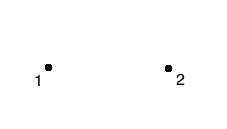(3) joining move: 1;2 (skipped) (skipped) 1,3;2,3 1,3,2,3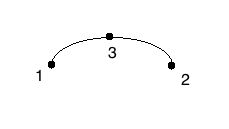(4) dividing move: 1,3,2,3 1,3,2,3,1 1,3,2/3,1 1,3,2/2,3,1 4,1,3,2/4,2,3,1 (skipped)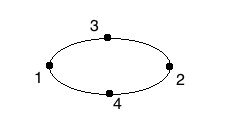(5) dividing move: 1,3,2,4/4,2,3,1 1,3,2,4,1/4,2,3,1 1,3,2,4/1/4,2,3,1 1,3,2,4/4,1/4,2,3,1 5,1,3,2,4/5,4,1/4,2,3,1 (skipped)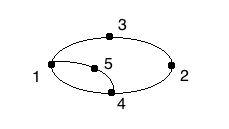(6) dividing move: 5,1,3,2,4/5,4,1/2,3,1,4 5,1,3,2,4/5,4,1/2,3,1,4,2 5,1,3,2,4/5,4,1/2,3/1,4,2 5,1,3,2,4/5,4,1/2,3/3,1,4,2 5,1,3,2,4/5,4,1/6,2,3/6,3,1,4,2 (skipped)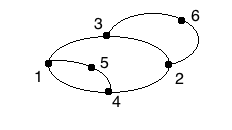### Analogy with graph

We have represented a plane graph using rows of stacks of cards.
 Playing card term Graph theory term ≈ card ≈ occurrence of vertex in boundary stack ≈ left-hand walk of vertices on boundary row ≈ face, i.e. set of boundaries table ≈ plane graph, i.e. set of faces

### Credits

Author: Josh Jordan
Initially published at http://www.wgosa.org/playingcardssprouts.htm

This document builds upon the sprouts notation system devised by Dan Hoey.

## Tuesday, April 23, 2013

### John Graham-Cunning's Minimum Coin Problems

In The Minimum Coin Problem, John Graham-Cunning poses the following problems:
1. Given a pile of coins and a target amount, find a way to pay exactly that amount using the maximum number of coins from the pile.
2. Given a pile of coins and a target amount, find a way to pay at least that amount using the maximum number of coins from the pile, such that removing any coin from the payment yields an amount that is less than the target amount.
3. Given a pile of coins — each type of coin having a given weight — and a target amount, find a way to pay at least that amount using the maximum weight of coins from the pile, such that removing any coin from the payment yields an amount that is less than the target amount.
These can each be posed as integer programming problems and solved with a solver such as GLPK. Here is a solution to problem 1, a solution to problem 2, and a solution to problem 3.